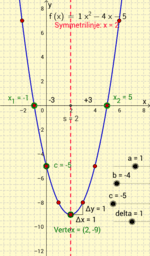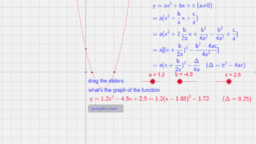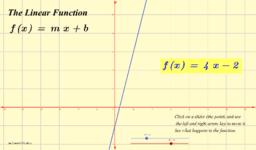Author:
Eve Chui
Chapter 3 Function and Graph Quadratic equation Constant, Linear and quadratic Equation
•The anatomy of a quadratic function

••